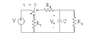# Simple ECE First Order Network

• Hashmeer

## Homework Statement

I need to resolve this network and find the equation for voltage at some time, t'>0. I know how to find the equation, but I'm having trouble finding the value for tau because I'm not sure what the equivalent resistance is.

tau = R*C

R1 = 4 k ohms
R2 = 7 k ohms
R3 = 2 k ohms
C = 101 microF

## The Attempt at a Solution

I've tried resolving the resistors in different ways, but I can't seem to figure out how they combine to find R equivalent.

I'm just looking for a hint as to how the total resistance can be found when t > 0.

Thanks!

#### Attachments

•HW Circuit.gif
1.1 KB · Views: 480

## Homework Statement

I need to resolve this network and find the equation for voltage at some time, t'>0. I know how to find the equation, but I'm having trouble finding the value for tau because I'm not sure what the equivalent resistance is.

tau = R*C

R1 = 4 k ohms
R2 = 7 k ohms
R3 = 2 k ohms
C = 101 microF

## The Attempt at a Solution

I've tried resolving the resistors in different ways, but I can't seem to figure out how they combine to find R equivalent.

I'm just looking for a hint as to how the total resistance can be found when t > 0.

Thanks!

On a problem like this, I would just write the KCL at the top node, including the I = C dv/dt term for the capacitor current, and solve the differential equation in exponential form. The term in the exponential will be the time constant tau.

There may be easier ways to solve it, but doing it with the KCL should always work, and avoid errors if you simplify the "equivalent resistance" incorrectly.

Thanks for the tip. I actually just made a units mistake... milliseconds not seconds.